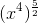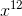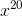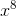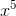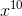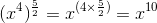# ISEE Middle Level Math : Algebraic Concepts

## Example Questions

### Example Question #71 : Algebra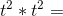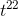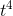Cannot be simplified further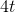Explanation:

Because the two terms have the same base, add the exponents and leave the base unchanged: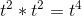Answer:### Example Question #71 : Algebra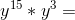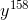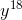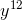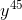Cannot be simplified furtherExplanation:

Add the exponents because we are multiplying two terms with the same base: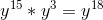Answer:### Example Question #41 : How To Multiply Variables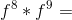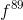Cannot be simplified further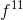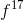Explanation: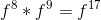Answer:### Example Question #71 : Algebra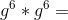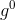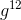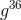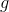Cannot be simplified furtherExplanation: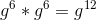Answer:### Example Question #41 : How To Multiply Variables

Multiply: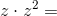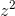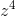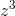Explanation: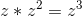Answer:### Example Question #41 : How To Multiply Variables

Multiply: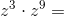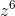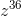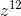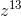Explanation: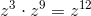Answer:### Example Question #71 : Algebra

Multiply: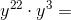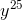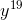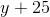Explanation: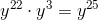Answer:### Example Question #42 : How To Multiply Variables

Multiply: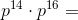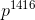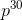Explanation: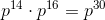Answer:### Example Question #43 : How To Multiply Variables

Simplify: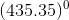The expression is undefined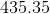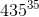Explanation:

Any non-zero number raised to the power of zero is equal to.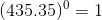### Example Question #41 : How To Multiply Variables

Simplify: